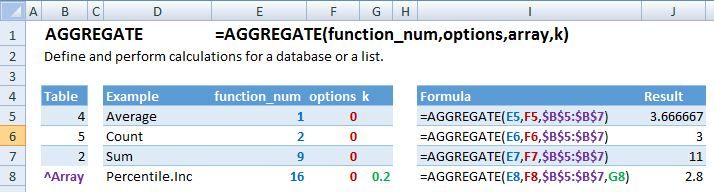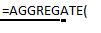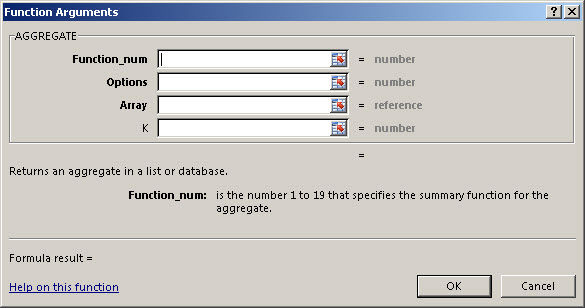### AGGREGATE Function – Examples in Excel, VBA, Google Sheets

This Excel Tutorial demonstrates how to use the Excel AGGREGATE Function in Excel to perform calculates to a database, with formula examples.

## AGGREGATE Function Description:

The AGGREGATE Function Calculates specified calculations (sum, average, etc.) for a database or a list.

## Formula Examples:Example Formula Result
Average =AGGREGATE(E5,F5,\$B\$5:\$B\$7) 3.66666666666667
Count =AGGREGATE(E6,F6,\$B\$5:\$B\$7) 3
Sum =AGGREGATE(E7,F7,\$B\$5:\$B\$7) 11
Percentile.Inc =AGGREGATE(E8,F8,\$B\$5:\$B\$7,G8) 2.8
AutoMacro - VBA Code Generator

## Syntax and Arguments:

The Syntax for the AGGREGATE Formula is:

`=AGGREGATE(function_num,options,array,k)`

### Function Arguments ( Inputs ):

function_num

options

array

k – 2 different options

## Additional Notes

Use the AGGREGATE Function to perform defined calculations on a database or list.

## AGGREGATE Examples in VBAYou can also use the AGGREGATE function in VBA. Type:
`Application.Worksheetfunction.Aggregate(function_num,options,array,k)`
For the function arguments (function_num, etc.), you can either enter them directly into the function, or define variables to use instead.

Return to the List of all Functions in Excel

## How to use the AGGREGATE Function in Excel:

To use the AND Excel Worksheet Function, type the following into a cell:
`=AND(`
After entering it in the cell, notice how the AND formula inputs appear below the cell:You will need to enter these inputs into the function. The function inputs are covered in more detail in the next section. However, if you ever need more help with the function, after typing “=AGGREGATE(” into a cell, without leaving the cell, use the shortcut CTRL + A (A for Arguments) to open the “Insert Function Dialog Box” for detailed instructions:For more information about the AGGREGATE Formula visit the
Microsoft Website.

## Excel Practice Worksheet

Practice Excel functions and formulas with our 100% free practice worksheets!

• Automatically Graded Exercises
• Learn Excel, Inside Excel!